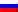# Distance measurements for ordinal attributes

Contenus

## Distance measurements for ordinal attributes

Many partitioning methods use distance measures to determine the similarity or dissimilarity between any pair of objects (such as Distance measures for ordinal attributes). It is common to designate the distance between two instances x_i and x_j as: d (x_i, x_j). A valid distance measurement must be symmetrical and obtain its minimum value (usually zero) in the case of identical vectors. The distance measure is called the metric distance measure if it also satisfies the following properties:

When attributes are ordinal, the sequence of values is important. In such cases, attributes can be treated as numeric attributes after mapping their range to [0,1]. This mapping can be done as follows:

where z_i, n is the normalized value of attribute a_n of object i. r_i, n is this value before normalization, and M_n is the upper limit of the domain of the a_n attribute (assuming the lower limit is 1).FRFRENRUES